# Lecture 25: Continuous problems¶

So far in this course we have studied discrete/finite-dimensional computations, concerning finite-dimensional vectors $\mathbf v \in \mathbb R^n$. We now turn our attention to continuous/infinite-dimensional computations, involving function $f : [-1,1] \rightarrow \mathbb R$. These are in some sense infinite-dimensional vectors, because a function can take a different value at infinitely many points. The goal of the remainder of the course is to solve continuous problems by reducing them to discrete problems.

We will see that there are continuous analogues of many discrete objects we have studied:

Discrete Continuous
Vectors $\mathbf v$ Functions $f(x)$
Summations $\sum_{k=1}^n v_k$ Integrals $\int_{-1}^1 f(x) dx$
Solving linear systems $A\mathbf x = \mathbf b$ Solving ODEs $u(0) = c, u'(t) = t u(t)$

We also have two classes of continuous problems: linear and nonlinear. Some nonlinear problems include:

1. Root Finding $f(x) = 0$ for smooth functions $f$
2. Nonlinear ODEs $u(0) = c, u'(t) = u(1-u)$

Finally, we will also consider the following more complicated linear problems:

1. Partial Differential Equations (PDEs): $u_t = u_{xx}, u(0,x) = u_0(x)$ (Heat equation)
2. Fourier expansions:
$$f(\theta) = \sum_{k=-\infty}^\infty \hat f_k e^{i k \theta}$$

for

$$\hat f_k \triangleq {1 \over 2 \pi} \int_0^{2\pi} f(\theta) e^{-i k \theta} d\theta$$

In this lecture, we will see that many of these tasks can be accomplished with inbuilt routines.

## Integration¶

Consider the problem of calculating an integral, that is, area underneath a curve. Let's take $f(x) = \sin x^2$ on $[-1,1]$:

In :
using PyPlot

f=x->sin(x.^2)  # anonymous function

x=linspace(-1.,1.,100)
plot(x,f(x));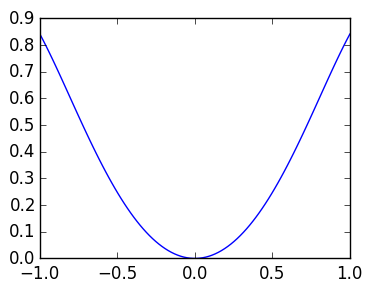We can use quadgk to calculate this integral, which returns the value (Q) and an error estimate (err):

In :
Q,err=quadgk(x->sin(x.^2),-1.,1.)

Out:
(0.6205366034467622,4.135691789031171e-12)

The speed of quadgk depends on the smoothness of the integrand, taking more time for oscillatory integrals:

In :
@time quadgk(x->sin(x.^2),-1.,1.)

  0.001603 seconds (352 allocations: 7.082 KB)

Out:
(0.6205366034467622,4.135691789031171e-12)
In :
@time quadgk(x->sin(100000x.^2),-1.,1.)

  0.738061 seconds (13.60 M allocations: 212.017 MB, 17.52% gc time)

Out:
(0.003973320903885958,5.919845667697868e-11)

## ODEs¶

Consider solving a simple scalar value ODE:

$$u(0) = a, u'(t) = t u(t)$$

for $t \in [0,1.]$. We can solve this using the ode45 routine in the ODE Package:

In :
using ODE

rhs=(t,u)-> -t*u   # the right-hand side of the ODE
a=0.5    # the inital condition

t,u=ode45(rhs,a,linspace(0.,10.,100))  # Returns a list of t and values u

plot(t,u)   # plots the solution;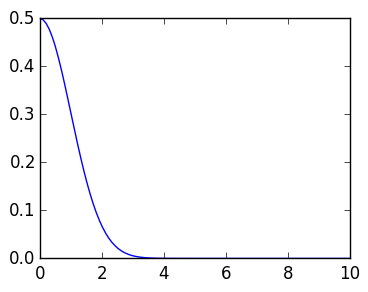For second order equations, we need to reduce it to a system of first order equations. Consider

$$u(0) = a, u'(0) = b, u''(t) = -t u(t)$$

We define a vector-value function containing $u$ and its derivative $u'$:

$$\mathbf u(t) \triangleq \begin{pmatrix} u(t) \cr u'(t) \end{pmatrix}$$

Therefore

$$\mathbf u'(t) = \begin{pmatrix} u'(t) \cr u''(t) \end{pmatrix}$$

So our original ODE is equivalent to the following system:

$$\mathbf u'(t) = \begin{pmatrix} u_2(t) \cr -tu_1(t) \end{pmatrix}$$

We can solve it by giving a Vector as the initial condition, and having the right-hand side function also returning a Vector:

In :
rhs=(t,u)->[u,-t*u]   # u is a Vector of same length as the initial condition
t,u=ode45(rhs,[a,b],linspace(0.,10.,100))
plot(t,u)  # plots both u and u;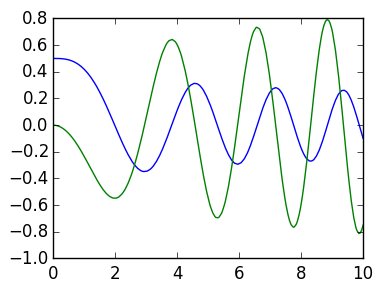We can use the Interact package to make ODE solving interactive:

In :
using Interact

f=figure()

@manipulate for a=0.:0.01:1.,b=0.:0.01:1.   # vary the initial conditions
withfig(f) do
rhs=(t,u)->[u,-t*u]
t,u=ode45(rhs,[a,b],linspace(0.,10.,100))
plot(t,u)
end
end

Out: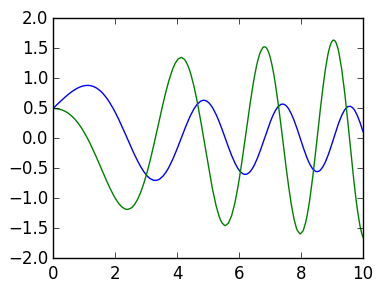The ODEs don't have to be linear: here is a solution to

$$u'' = u^2 - t u$$
In :
using Interact

f=figure()

@manipulate for a=0.:0.01:1.,b=0.:0.01:1.
withfig(f) do
rhs=(t,u)->[u,u^2-t*u]
t,u=ode45(rhs,[a,b],linspace(0.,10.,100))
plot(t,u)
axis([t,t[end],-10.,10.])
end
end

Out: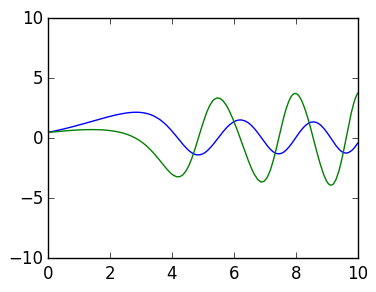## Stiff ODEs¶

Consider $\mathbf u' = A \mathbf u$, $\mathbf u(0) = [1.,0.]$. The equation becomes "stiff" as eigenvalues of A become large, and so it is faster to use ode23s since it has an s in its name:

In :
A=[0 1; -10000 -10001]

@time ode45((t,u)->A*u,[1,0.],linspace(0.,10.,100))
@time ode23s((t,u)->A*u,[1,0.],linspace(0.,10.,100))

eigvals(A)

  0.163711 seconds (2.45 M allocations: 68.955 MB, 10.34% gc time)
0.007875 seconds (37.35 k allocations: 3.401 MB)

Out:
2-element Array{Float64,1}:
-1.0
-10000.0

Timing is not the only thing that's important, we also are concerned with accuracy. Turns out ode45 is the most accurate though slowest, while ode4s returns nonsense:

In :
A=[0 1; -10000 -10001]

ex=expm(A*10.)*[1,0.]
t,u=ode45((t,u)->A*u,[1,0.],linspace(0.,10.,100))
println(norm(u[end]-ex))
t,u=ode23s((t,u)->A*u,[1,0.],linspace(0.,10.,100))
println(norm(u[end]-ex))

2.1881576804437884e-11
9.842153036119409e-8
7.295253980841093e-10


# FFT¶

The FFT lets us recover Fourier modes from there samples at evenly spaced points. Here is a plot of the points where we will sample the function:

In :
n=10
θ=linspace(0.,2π*(1-1/n),n)

plot(θ,zeros(n);marker=".",linestyle="")
axis([0,2π,-0.1,0.1]);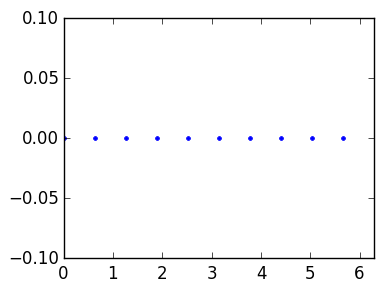We can sample a single Fourier mode at this grid and plot its real and imaginary parts:

In :
vals=exp(im*θ)
plot(θ,real(vals))
plot(θ,imag(vals));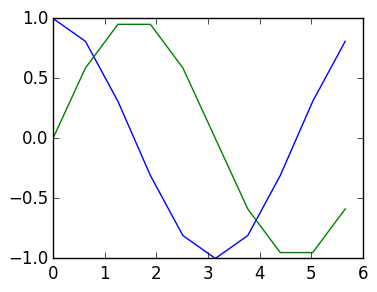The FFT gives a map from the values back to the modes:

In :
n=10
θ=linspace(0.,2π*(1-1/n),n)

vals=exp(3*im*θ)+2exp(4*im*θ)

real(fft(vals)/n  )

Out:
10-element Array{Float64,1}:
0.0
-2.17353e-18
-2.62106e-16
1.0
2.0
2.88658e-16
1.77636e-16
0.0
-9.31649e-17
-8.66443e-17

Negative modes show up at the end of the vector:

In :
n=10
θ=linspace(0.,2π*(1-1/n),n)

vals=exp(-im*θ)

real(fft(vals)/n)

Out:
10-element Array{Float64,1}:
-2.22045e-17
0.0
-3.15391e-18
-4.44089e-17
-1.9913e-17
0.0
6.1899e-18
-4.44089e-17
3.90815e-17
1.0        

Sampling a sum of Fourier modes at the grid can also be accomplished using the inverse FFT:

In :
cfs=fft(vals)/n

norm(ifft(cfs)*n-vals)

Out:
4.611102534756203e-16

We will go into more details later.